Previous: Editing Text Areas  Up: Selecting and Editing Objects  Next: Text Outlines

# Combining a Text Area and Path to Form a Text-Path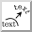A text area and a path can be combined to form a text-path. The underlying path will not be visible (except in path edit mode) and the text will run along the path. If the original text area was in outline mode, the new text-path will also be in outline mode, possibly with a fill colour, depending on the original text area and path fill colours. (If the original text area didn't have a fill colour, the path fill colour will be applied.)

The horizontal anchor determines whether the text should start at the first control point of the underlying path or if it should be centred along the path or if it should be right aligned at the end control point. The vertical anchor determines whether the base, bottom, top or middle of the text should be aligned on the path. However, the vertical anchor isn't implemented by the LaTeX export functions. Note that if the text is longer than the path, the text will be truncated.

For example, the text area and path in Figure 8.12(a) are combined to form a text-path. The original text area's horizontal anchor was set to left, so the text along the path starts at the first control point in Figure 8.12(b). In Figure 8.12(c) the horizontal anchor has been changed to centre, and in Figure 8.12(c) the horizontal anchor has been changed to right.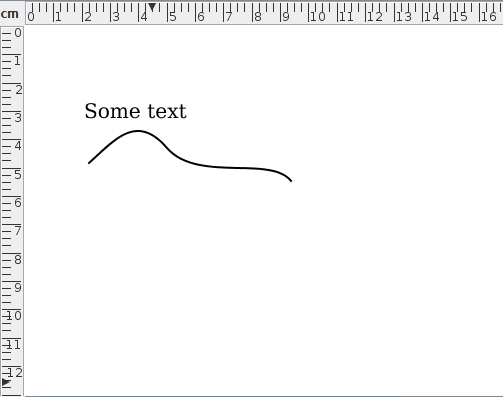(a)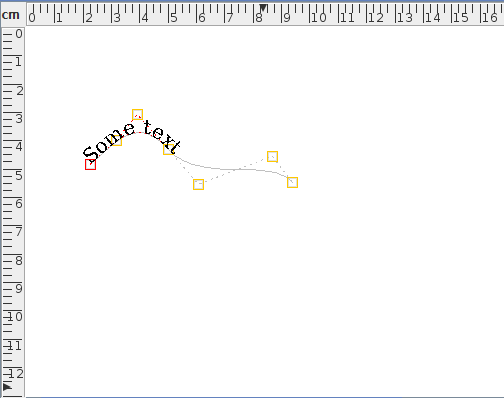(b)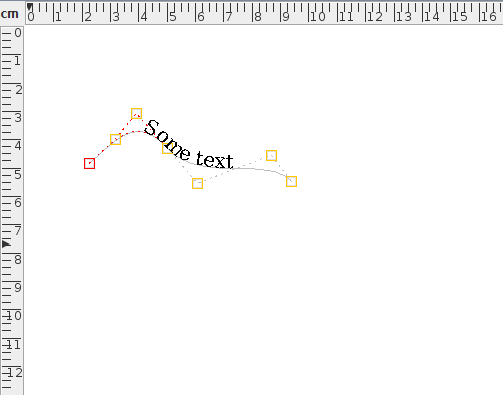(c)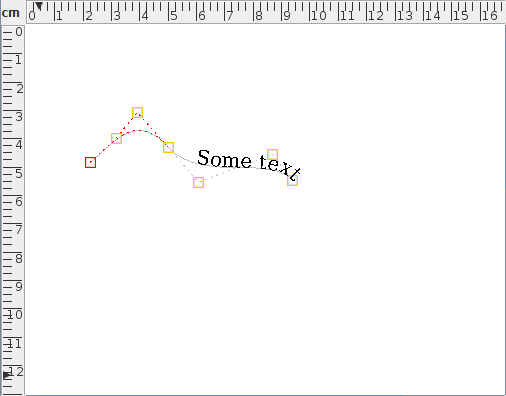(d)
Figure 8.12: Combining a text area and path to form a text-path: (a) the original text area and path; (b) the resulting text-path with left horizontal anchor; (c) centred anchor; (d) right anchor. (The text-paths are in edit mode to show the underlying path.)

Once a path has been combined with a text area, the path line style attributes are lost as the path is only used as a guide to position the text. Most path functions, such as path union, are applied to the underlying path and the text is adjusted to follow the new path. Transformations using the rotate, scale and shear functions are applied to the underlying path not the text. You can either transform the text using the transformation functions before combining it with a path or transform it after combining by changing the text transformation matrix.

Note there is a difference between applying symmetry to a text-path and converting a text area and symmetric shape to a text-path. For example, consider the text area and path in Figure 8.13(a). If you first combine them to form a text-path (Figure 8.13(b)) and then add symmetry (Figure 8.13(c)), the result is a text-path where the text is reflected across the line of symmetry. Conversely, applying symmetry to the path first (Figure 8.13(d)) and then combining with the text area yields a text area where only the underlying path has symmetry (Figure 8.13(e)).

A similar effect applies with other types of composite shapes.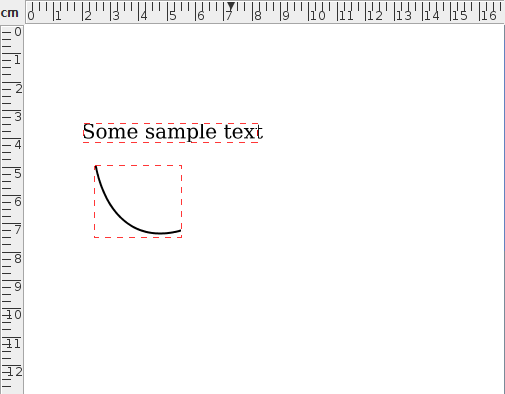(a)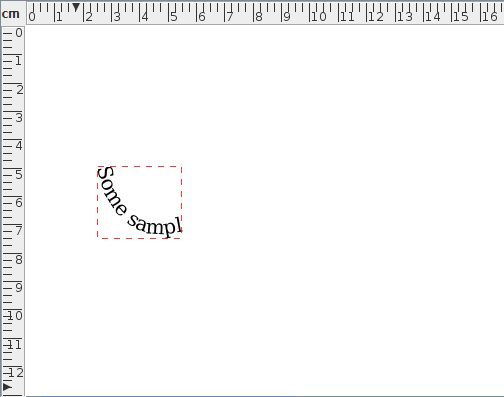(b)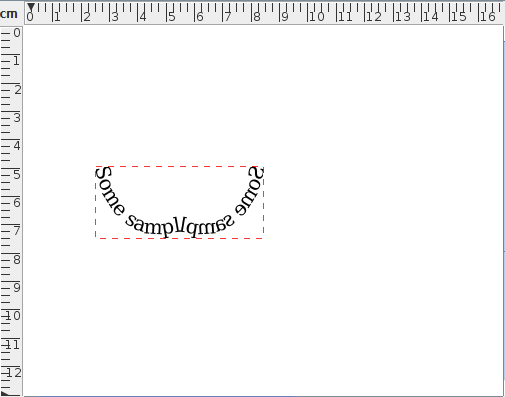(c)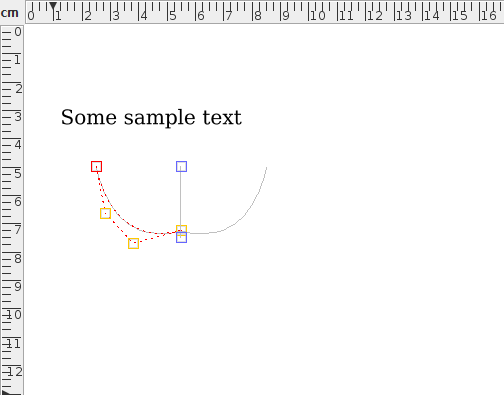(d)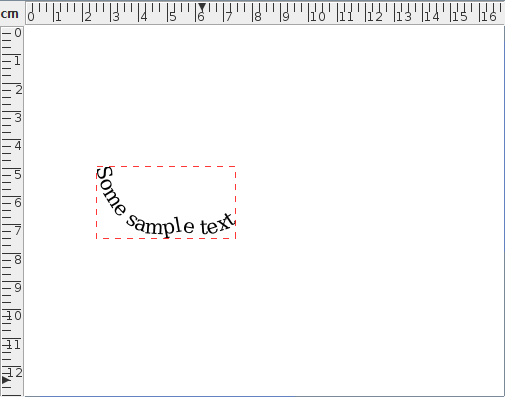(e)
Figure 8.13: Symmetric text-paths: (a) original text area and path; (b) text area and path in (a) have been combined to form a text-path; (c) the text-path in (b) has had symmetry applied to it in edit path mode; (d) the path in (a) has had symmetry applied to it; (e) the text area and symmetric path in (d) have been combined to form a text-path.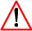Restrictions apply to text-paths with the export functions as some text-path effects aren't emulated in LaTeX, such as the vertical anchor, gradient paint and outlines. You can determine the behaviour on export via the TeX Configuration settings.